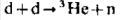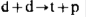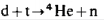# Here are the formulas of the reactions which are In the basis of a controlled thermonuclear fusion

 Reaction: Verification: H21 + H21 → He32 + n10 45 + 45 → 57 + 33 90=90Reaction: Verification: H21 + H21 → H31 + p11 45 + 45 → 78 + 12 90=90Reaction: Verification: H21 + H31 → He42 + n10 45 + 78 → 90 + 33 123=123References: Прохоров А.М. Физическая энциклопедия в 5-ти томах. Том 5. [Prohorov, A.M. Physical Encyclopedia in 5 volumes. Vol. 5] Moscow: Soviet Encyclopedia, 1988. P. 104 [In Russian].